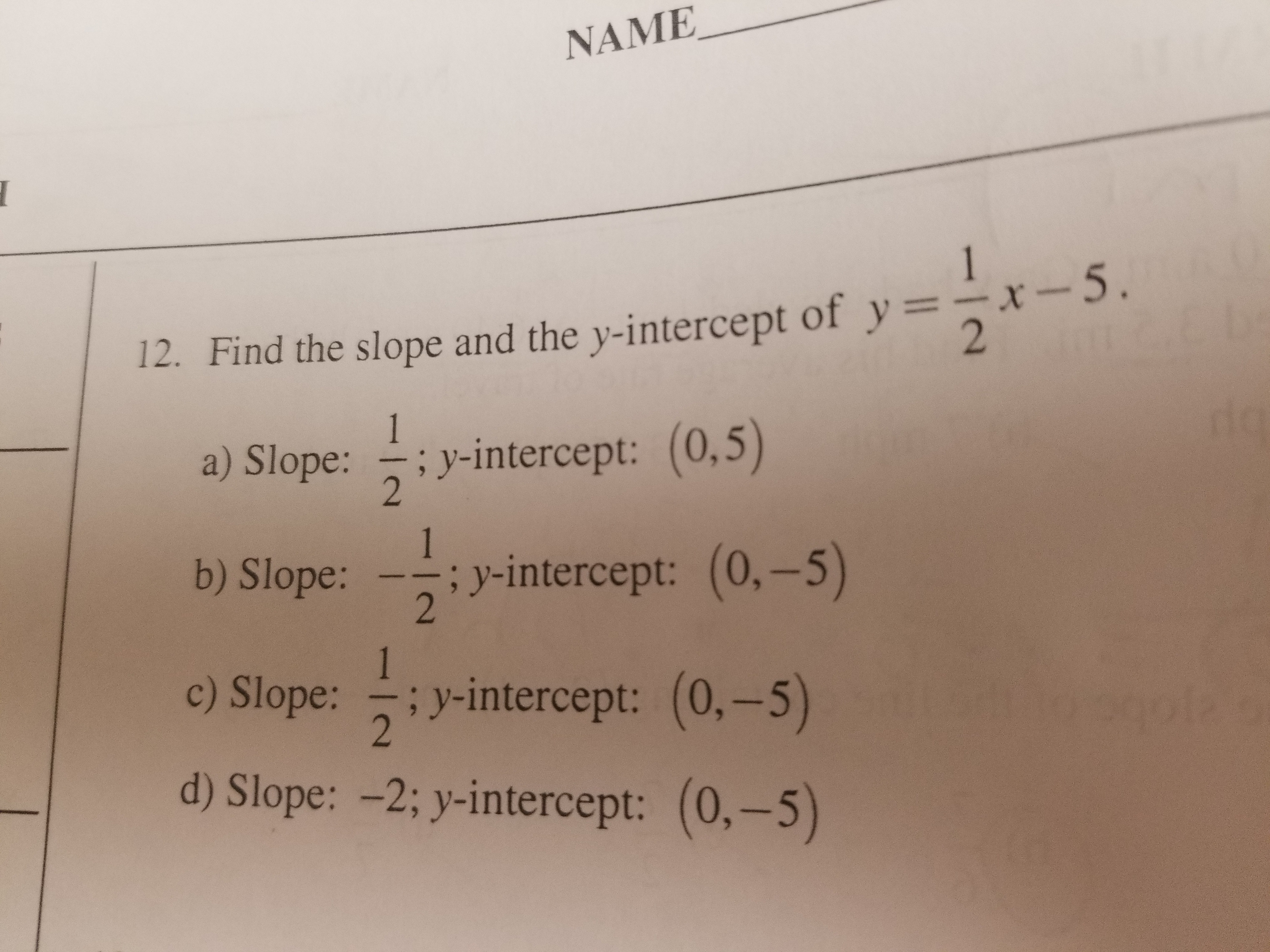# NAME12. Find the slope and the y-intercept of y =-x-5a) Slope:y-intercept: (0,54: y-intercept (0.-5)b) Slope:--;inteo:-intercept: (0.-5)d) Slope: -2; y intercept: (0,-5)

Question
1 viewshelp_outlineImage TranscriptioncloseNAME 12. Find the slope and the y-intercept of y =-x-5 a) Slope: y-intercept: (0,5 4: y-intercept (0.-5) b) Slope: --; inte o:-intercept: (0.-5) d) Slope: -2; y intercept: (0,-5) fullscreen
check_circle

Step 1

Calculation:

The given linear function is y = 1/2 (x) – 5.

The y intercept of the above function can be obtain by equating x = 0 as follows.

Step 2

The general slope intercept form of a linear equation is y = mx + b, where m is a slope and b is y intercept.

Compare th...

### Want to see the full answer?

See Solution

#### Want to see this answer and more?

Solutions are written by subject experts who are available 24/7. Questions are typically answered within 1 hour.*

See Solution
*Response times may vary by subject and question.
Tagged in

### Other